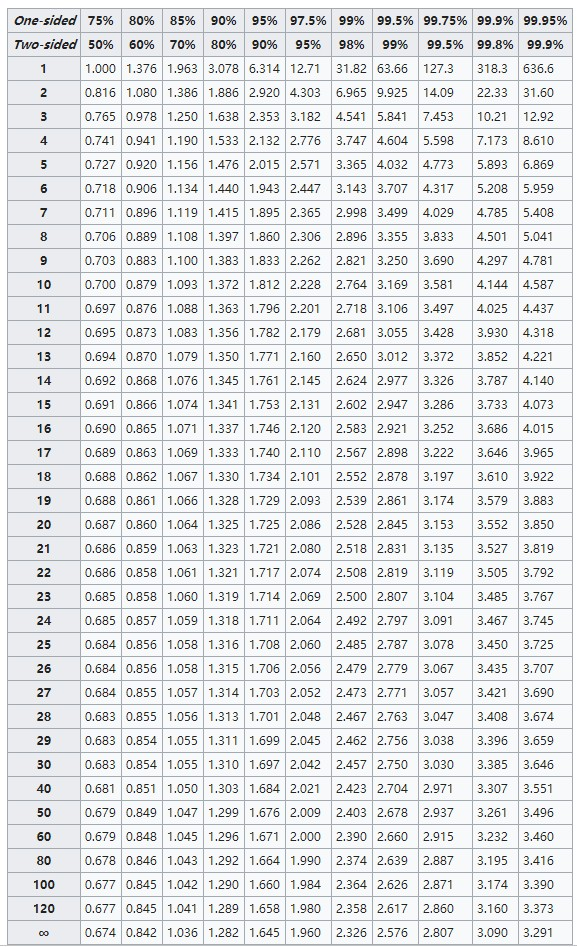# 假设检验

1. 提出原假设 $H_0$ 和备择假设 $H_1$
2. 考虑检验中对样本做出的统计假设；例如，关于独立性的假设或关于观测数据的分布的形式的假设
3. 确定适当的检验统计量 $T$
4. 在零假设下推导检验统计量的分布。在标准情况下应该会得出一个熟知的结果。比如检验统计量可能会符合t-分布正态分布
5. 选择一个显著性水平 $\alpha$，若低于这个概率阈值，就会拒绝零假设。常用 5% 和 1%
6. 根据在零假设成立时的检验统计量 $T$ 分布，找到数值最接近备择假设，且几率为显著性水平 $\alpha$ 的区域，此区域称为“拒绝域”，意思是在零假设成立的前提下，落在拒绝域的几率只有 $\alpha$
7. 针对检验统计量 $T$，根据样本计算 / 查表得到其估计值 $t_{obs}$$p-value$
8. 若估计值 $t_{obs}$ 落在“拒绝域”（如 $p < \alpha$），接受对立假设；反之接受零假设

## ANOVA F-test

ANalysis Of VAriance，方差分析，又称变异数分析，F-test。

## T-test

t-test 改进了 z-test 在样本量小于 30 时误差大的问题。

$\overline{X}_n = \frac{\sum_i^n X_i}{n}$

$S_n = \frac{1}{n-1}\sum_i^n (X_i - \overline X)^2$

$\frac{\overline X_n - \mu_0}{\sigma / \sqrt n}$

$t = \frac{\overline X_n - \mu_0}{S_n / \sqrt n}$

$t = \frac{\overline X_1 - \overline X_2}{S_{\overline X_1 - \overline X_2}}$

For example, given a sample with a sample variance 2 and sample mean of 10, taken from a sample set of 11 (10 degrees of freedom), using the formula

$\overline X \pm t_{\alpha, n-1}\cdot \frac{S_n}{n}$

we can determine that at 90% confidence, we have a true mean lying below 10.58 and over 9.41.

In other words, on average, 80% of the times that upper and lower thresholds are calculated by this method, the true mean is both below the upper threshold and above the lower threshold. This is not the same thing as saying that there is an 80% probability that the true mean lies between a particular pair of upper and lower thresholds that have been calculated by this method.

## 最小样本数估算

$p'-z \sqrt{\frac{p'(1-p')}{n'}}\le p\le p'+z \sqrt{\frac{p'(1-p')}{n'}}$

https://www.stat.ubc.ca/~rollin/stats/ssize/b1.html

Statistical Power （$1 -\beta$）统计学功效：在假设检验中， 拒绝原假设后， 接受正确的替换假设的概率。在假设检验中有 $\alpha$ 错误和 $\beta$ 错误。$\alpha$ 错误是 FP 错误， $\beta$ 错误是 FN 错误。

Significiant Level 显著性水平 $\alpha$。同时也是 Type I Error 出现的概率，FP，第一类错误意味着新的产品对业务其实没有提升，我们却错误的认为有提升。

Minimum Detectable Effect 最小改善程度 $\delta = (t_{\alpha/2} + t_{\beta} \sigma \sqrt{2/n})$

$n \approx 16 \frac{\sigma^2}{\delta^2}$

https://jeffshow.com/caculate-abtest-required-sample-size.html#more-1798

https://www.cnstat.org/samplesize/11/

http://www.evanmiller.org/how-not-to-run-an-ab-test.html

http://www.evanmiller.org/ab-testing/sample-size.html

https://zhuanlan.zhihu.com/p/40919260

## P-value

p 值是指在一个概率模型中，统计摘要（如两组样本均值差）与实际观测数据相同，或甚至更大这一事件发生的概率 。换言之，是检验假设H0成立或表现更严重的可能性。p值若与选定显著性水平（0.05或0.01）相比更小，则H0会被否定而不可接受。然而这并不直接表明原假设正确。通常在H0设下，p值是一个服从[0,1]区间均匀分布随机变量，在实际使用中因样本等各种因素存在不确定性。产生的结果可能会带来争议。

## 效应量

Effect size d r

## 参数估计：正态总体

• 点估计（矩法 / 极大似然法）：求得参数
• 区间估计：求置信系数为 p 的参数区间

## 参考

https://www.zhihu.com/question/309884517

## $t_{\alpha,n-1}$

t-统计量表## $Z_{\alpha/2}$

https://www.statisticshowto.datasciencecentral.com/z-alpha2-za2/